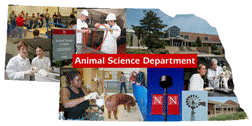## Animal Science Department## Faculty Papers and Publications in Animal Science

#### Date of this Version

January 1992

Published in J. Anim. Sci. 1992. 70:57-61.

#### Abstract

A data set that was used to estimate covariance components with REML for an animal model with eight measures of ovulation rate treated as separate traits was used as a template to simulate data sets of eight multivariate normal traits that were then truncated to binomial traits. The model for simulation included eight measures on 610 animals with 1,071 animals in the numerator relationship matrix. Heritabilities were equal for the eight measures, and both genetic and phenotypic correlations among the measures were equal. Ten replications for each combination of heritability (.15, .25, and .35) and genetic correlation (.50, .66 and .90) were simulated on the normal scale. For each replicate, estimates of the eight heritabilities and 28 genetic correlations were obtained by multiple-trait REML. The usual transformation of heritability estimated on the binomial scale overestimated heritability on the normal scale. Genetic correlations on the binomial scale seriously underestimated the correlations on the normal scale. Standard errors of the estimates obtained by replication were somewhat larger than the approximate SE from REMLPK (the multitrait REML program of K. Meyer). A final set of 10 simulated replications with heritability of .25 and genetic correlation of 1.00 resulted in average estimates of .18 for heritability and of .66 for genetic correlation that agree closely with those from the analysis of measures of ovulation at eight estrous cycles used as a template; averages for heritability of .l6 and for genetic correlation of .66 were obtained .

COinS